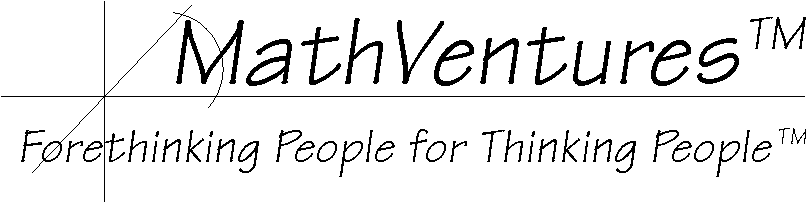# Logic  the Art of Reasoning

Mathematics   the Art of Studying Patterns and Relationships Using Logic# Subtraction without Borrowing

No borrowing, no negative numbers, this simple method makes subtraction easy.

The standard multi-digit subtraction procedure (algorithm) that is taught to most, if not all, students requires that the student is capable of subtracting any single-digit number from any number in the range of 10 to 19. For the student must be able to "borrow" a "ten" from the digit to the left of the current one. In other words, the strongest demand on the student's mathematical skill is the ability to mentally subtract any single digit number from any number between 10 and 19, inclusively.

For the students who struggle with this requirement this new procedure provides an easier alternative. It required that the strongest subtraction skill is the ability to subtract any single-digit number from any single-digit number. It also requires the ability to add multi-digit numbers, a skill that students are assumed to possess before they are taught multi-digit subtraction.

This procedure does not employ borrowing. Instead it replaces the source number with a substitute from which the subtraction can proceed without borrowing. After the subtraction is finished, the result is adjusted by adding the number that was used to generate the substitute source number.

A bonus of this procedure is the fact the actual subtraction can proceed from left to right just as traditionally it is done from right to left. In fact, as long as digits are subtracted in their columns and subtraction is carried out in each and every column, the order is no longer relevant.

Subtraction Without Borrowing, a Simple ExampleCopyright  © 1993-2008 Ten Ninety, All Rights Reserved
Last Update: Sep. 1, 2008